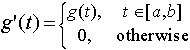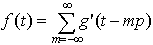EECS20N: Signals and Systems

# Periodic and finite signals

For signals where the domain is Time, Reals, Integers, or any other set that contains the sum of any two elements of the set, we can define a periodic signal. Assuming the domain is Time, a periodic signal f with period pTime is one where for all tTime

f(t) = f(t + p).

For example, the sinusoidal signal where for all tTime

f(t) = sin(ω 0t)

is a periodic signal with period 2π /ω 0. A finite signal in time is a signal whose domain is a subset [a,b] ⊂ Time, for some finite a and b. For example, the signal g where for all t ∈ [0, 2π /ω 0] ⊂ Time,

g(t) = sin(ω 0t)

is a finite signal with duration 2π /ω 0.

A finite signal with duration p can be used to define a periodic signal with period p. All that is needed is to periodically repeat the finite signal. Formally, given a finite signal g: [a,b] → Reals, we can define a signal g': Reals → Reals with an augmented domain byIn other words, g'(t) is simply g(t) inside its domain, and zero elsewhere. Then with the examples above,where p = b − a. That is, the periodic signal is just a sum of all versions of g(t) that have been shifted in time by multiples of p. Thus, a periodic signal can be defined in terms of a finite signal, which represents one period, and a finite signal can be defined in terms of a periodic signal (by taking one period).

We will see that any periodic signal, and hence any finite signal, can be described as a sum of sinusoidal signals. This result, known as the Fourier series, is one of the fundamental tools in electrical engineering.

# Exercises

1. Define finite and periodic for images.
2. Define finite and periodic for discrete-time signals, where the domain is Integers.